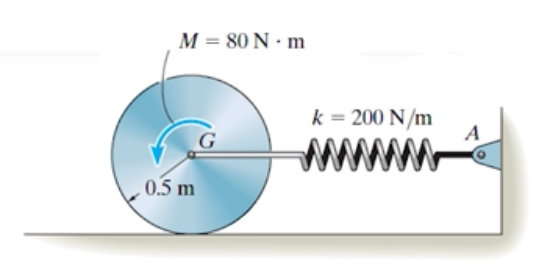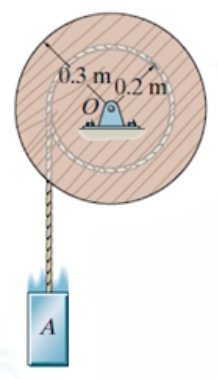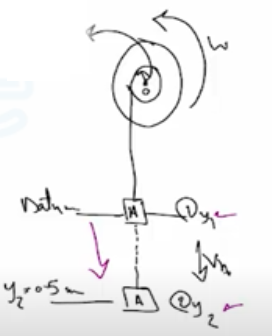Need Help?

Subscribe to Dynamic

###### \${selected_topic_name}
• Notes

$\begin{array}{l}{\text { 18-25. The } 30 \text { -kg disk is originally at rest, and the spring is }} \\ {\text { unstretched. A couple moment } M=80 \mathrm{N} \cdot \mathrm{m} \text { is then applied }} \\ {\text { to the disk as shown. Determine how far the center of mass }} \\ {\text { of the disk travels along the plane before it momentarily }} \\ {\text { stops. The disk rolls without slipping. }}\end{array}$$m=30 \mathrm{kg}$

$M=80 \mathrm{N} \cdot \mathrm{m}$

$\text { kinetic energy }$

$T_{1}=T_{2}=0$

$\theta=\frac{S_{G}}{r}=\frac{S_{G}}{0.5}=2 S_{G}$

$U_{M}=M \theta=80(2S_G) \mathrm{s} 160 \mathrm{S}_{G}$

$U_{F{sp}}=-\frac{1}{2} k x^{2}=-\frac{1}{2} 200\left(S_ G^{2}\right)=-100{S}_ G^{2}$

$T_{1}+\Sigma U_{1 \rightarrow 2}=T_{2} \Rightarrow 0+\left(160S_G-100 S_G^{2}\right)=0$

$160S_G-100S_G^{2}=0$

$S_G(160-100S_G)=0$

$160-100S_G=0$

$S_{G}=1.6 \mathrm{m}$

$\begin{array}{l}{\text { 18-39. The spool has a mass of } 50 \mathrm{kg} \text { and a radius of gyration }} \\ {\text { of } k_{O}=0.280 \mathrm{m} \text { . If the } 20-\mathrm{kg} \text { block } A \text { is released from rest, }} \\ {\text { determine the velocity of the block when it descends } 0.5 \mathrm{m} .}\end{array}$$m_ s=50 k g$

$k_{0}=0.28 \mathrm{m}$

$m _b=20 k g$

$s=0.5 \mathrm{m}$$v_{1}=\left(v_{g}\right)_1=w_{A} y_{1}=20(9.81) 0=0$

$v_{2}=\left(v_{g}\right)_{1}=-w_{A} y_{2}=-20(9.81)(0.5)=-98.1 \mathrm{J}$

$\omega=\frac{v_{A}}{r_{A}}=\frac{v_{A}}{0.2}=5 v_{A}$

$I_{0}=m k_0^{2}=5{0}(0.28)^{2}=3.92 \mathrm{kg}.\mathrm{m}^{2}$

$T=\frac{1}{2} I_{0} \omega^{2}+\frac{1}{2} m_{A} V_{A}^{2}$

$=\frac{1}{2}(3.92)(5 v_A)^{2}+\frac{1}{2}(20) v_{A}^{2}$

$=59 V_{A}^{2}$

$T_{1}=0$

$T_{1}+v_{1}=T_{2}+v_{2}$

$0+0=59 v _A^{2}+(-98.1)$

$V_{A}=1.289 \simeq 1.29 \mathrm{m} / \mathrm{s}$